# 第十四，十五周作业

1.实验代码

#include<stdio.h>
int main()
{
int i,sum=0;
int a;
for(i = 0;i < 10;i++)
{
scanf("%d" , &a[i]);
}
for(i = 0;i < 10;i++)
{
if(a[i]%2==0){
sum=sum+a[i];}
}
printf("s=%d",sum);
return 0;
}

2.设计思路
(1)主要描述题目算法

(2)流程图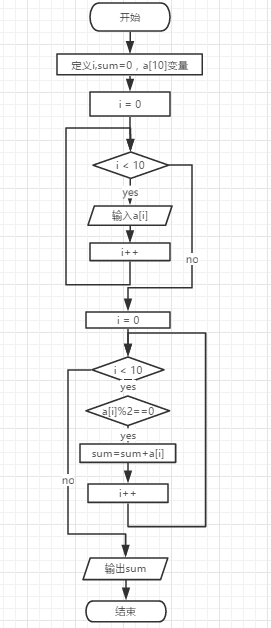3.本题调试过程碰到问题及解决办法

错误提示截图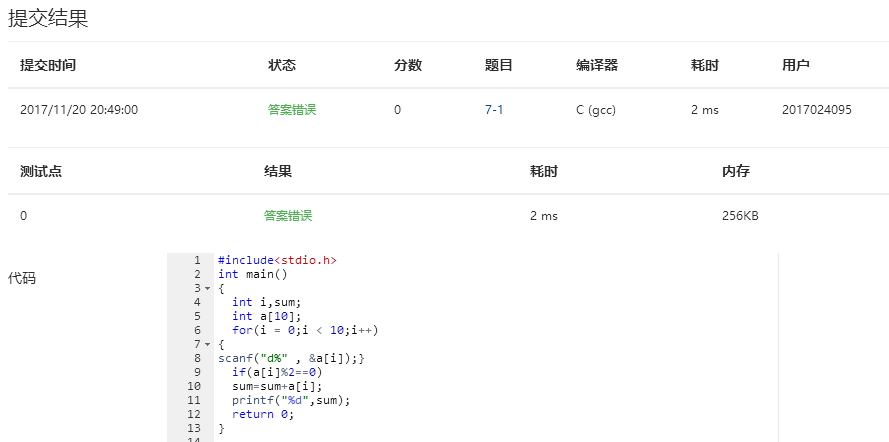4.本题PTA提交列表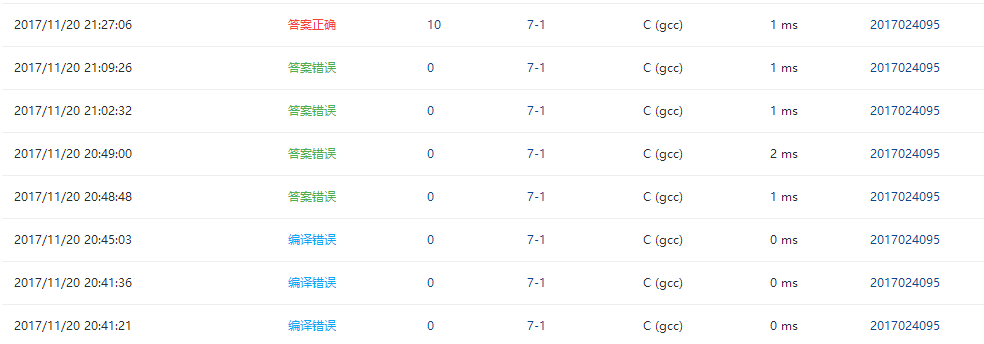1.实验代码

#include<stdio.h>
int main()
{
int i,sum=0;
int a;
double average;
for(i = 0;i < 10;i++)
{
scanf("%d" , &a[i]);
sum=sum+a[i];
}
average=sum/10;
for(i = 0;i < 10;i++)
{
if(a[i]<average)
printf("%d ",a[i]);
}
printf("\n");
return 0;
}

2.设计思路
(1)主要描述题目算法

(2)流程图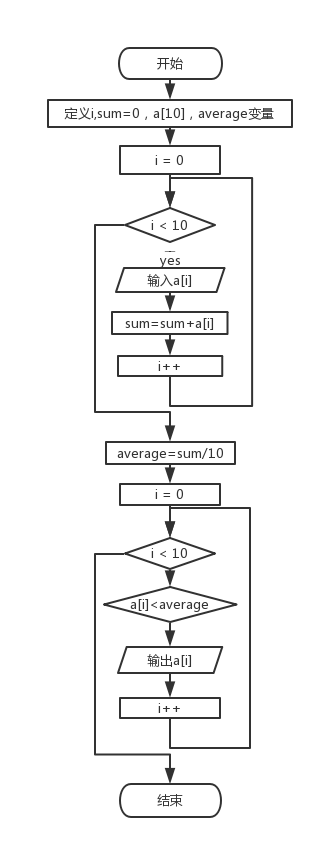3.本题调试过程碰到问题及解决办法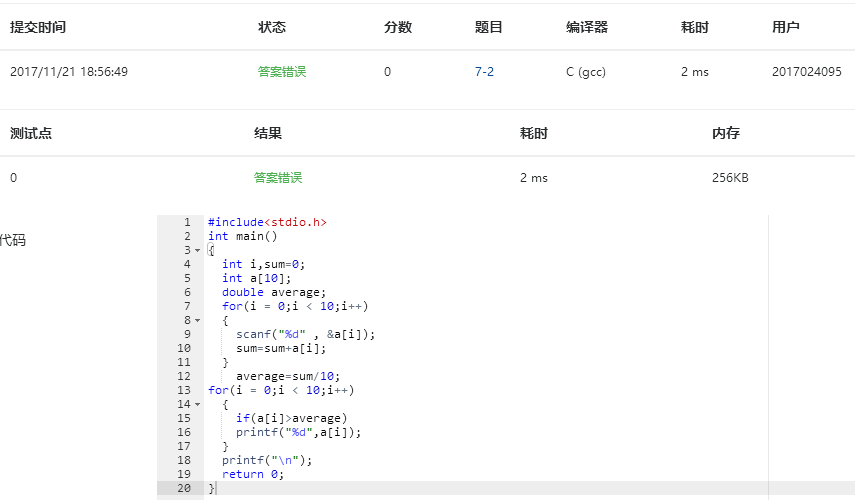4.本题PTA提交列表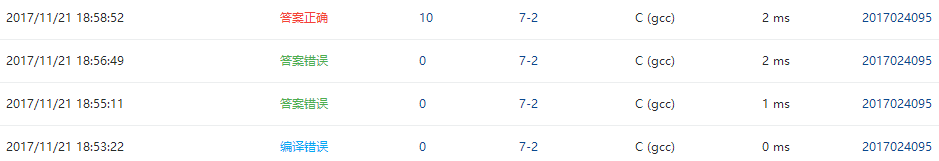1.实验代码

#include <stdio.h>
int main( )
{

int i;
int a={1, 1};
printf ("%6d",a);
printf ("%6d",a);
for (i=2;i<12;i++)
{
a[i]=a[i-2]+a[i-1];
printf ("%6d",a[i]);
if ((i+1)%3==0)
{
printf ("\n");}
}
return 0;
}

2.设计思路
(1)主要描述题目算法

(2)流程图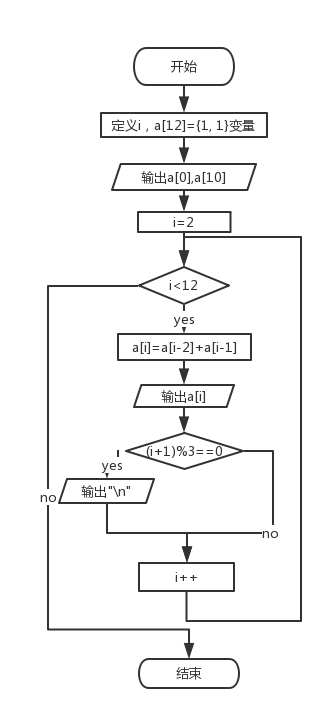3.本题调试过程碰到问题及解决办法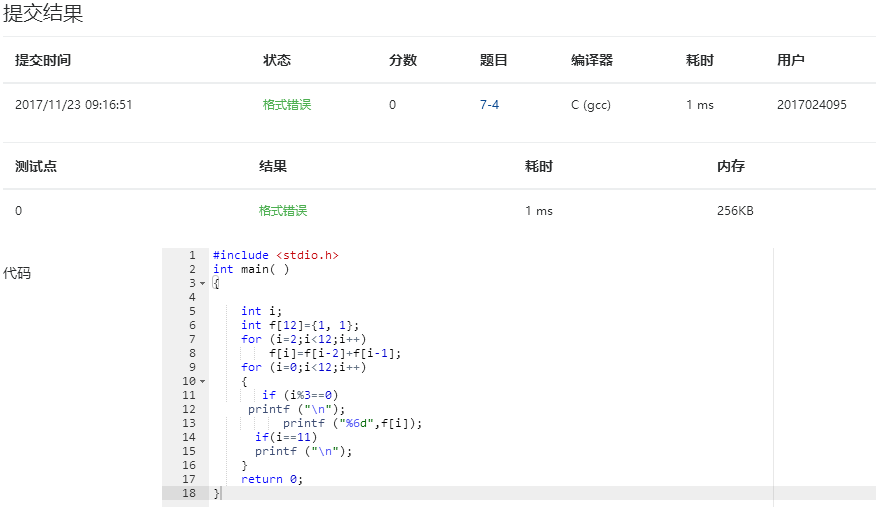4.本题PTA提交列表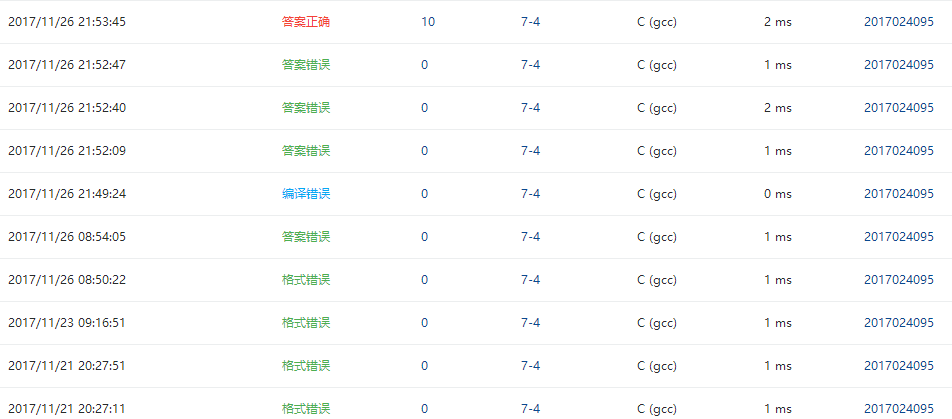1.实验代码

#include<stdio.h>
int main()
{
int n,i,index;
scanf("%d",&n);
int a[n];
for(i=0;i<n;i++)
{
scanf("%d ",&a[i]);
}
index=0;
for(i=1;i<n;i++)
{
if(a[i]>a[index]){index=i;}
}
printf("%d %d",a[index],index);
return 0;
}

2.设计思路
(1)主要描述题目算法

(2)流程图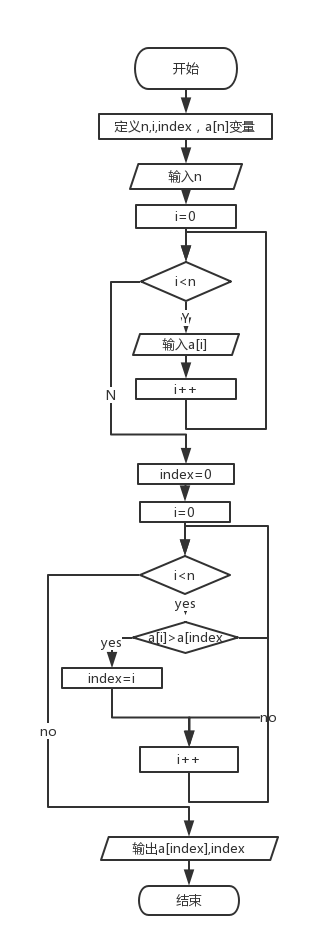3.本题调试过程碰到问题及解决办法4.本题PTA提交列表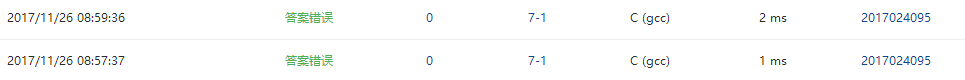1.实验代码

#include<stdio.h>
int main()
{
int i,k,a,n,temp,max;
scanf("%d",&n);
for(i=0;i<n;i++){
scanf("%d",&a[i]);
}
for(k=0;k<n-1;k++){
max=k;
for(i=k+1;i<n;i++)
if(a[max]<a[i])
max=i;
temp=a[max];
a[max]=a[k];
a[k]=temp;
}
for(i=0;i<n;i++){
if(i+1==n)
printf("%d",a[i]);
else
printf("%d ",a[i]);
}
return 0;
}

2.设计思路
(1)主要描述题目算法

(2)流程图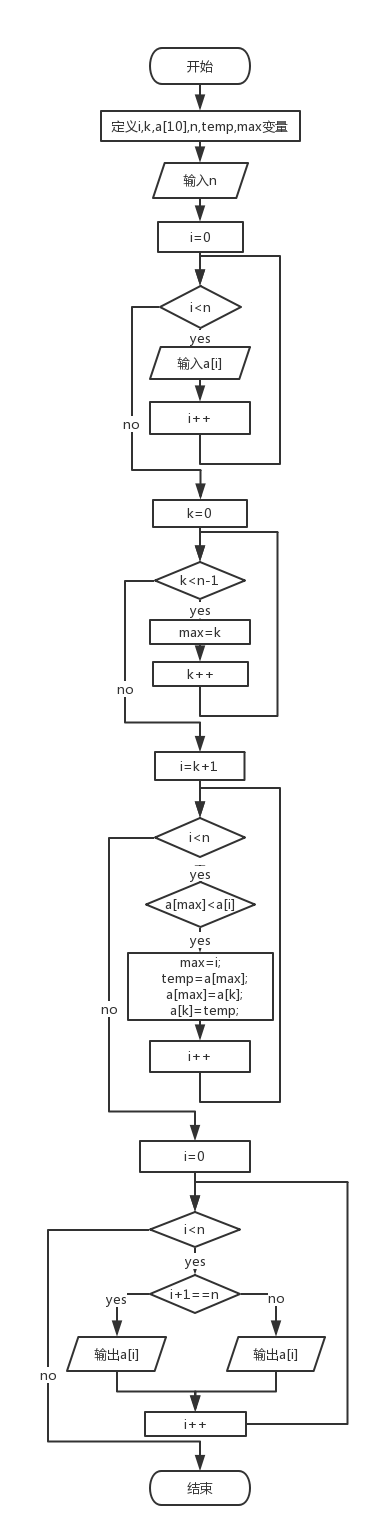3.本题调试过程碰到问题及解决办法
错误提示截图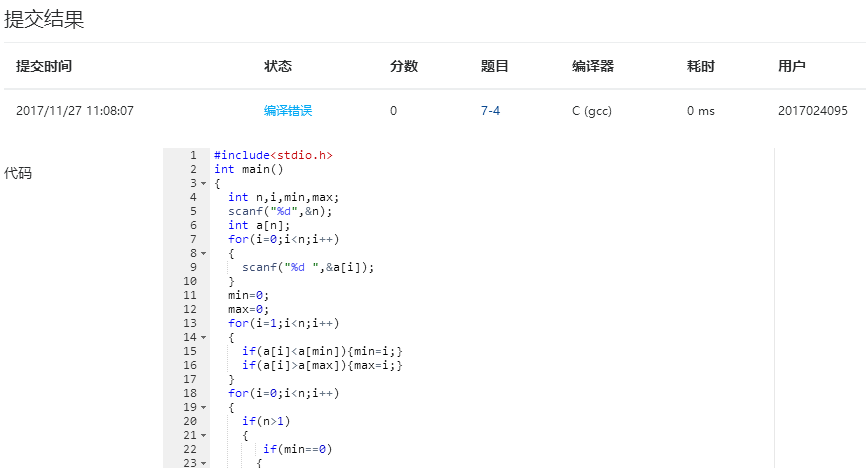4.本题PTA提交列表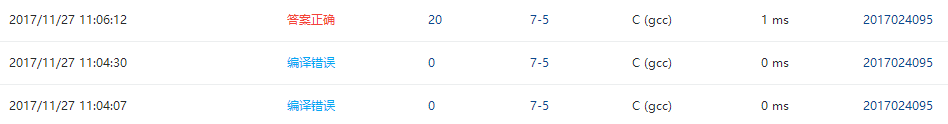1.实验代码

#include <stdio.h>
#define M 10
#define N 10

int main()
{
int a[M][N];
int sum[M];
int m,n,i,j;

scanf("%d %d",&m,&n);

for(i=0;i<m;i++)
{
sum[i]=0;
for(j=0;j<n;j++)
{
scanf("%d",&a[i][j]);
sum[i]+=a[i][j];
}
}

for(i=0;i<m;i++)
{
printf("%d\n",sum[i]);
}

return 0;
}

2.设计思路
(1)主要描述题目算法

(2)流程图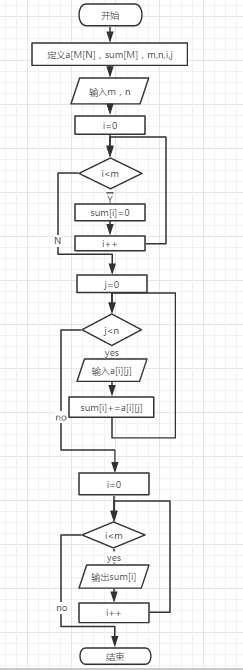3.本题调试过程碰到问题及解决办法

错误提示截图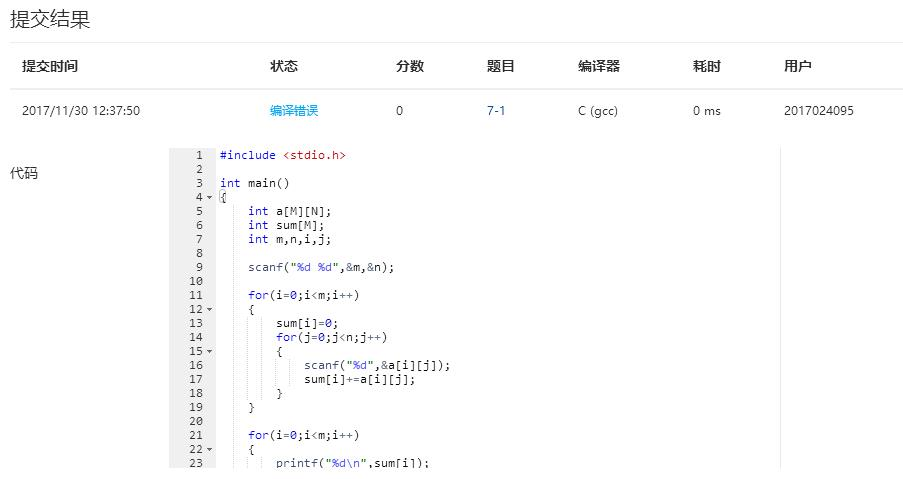4.本题PTA提交列表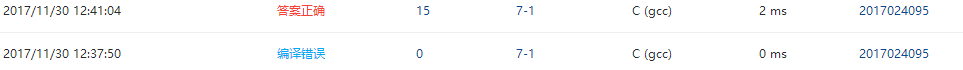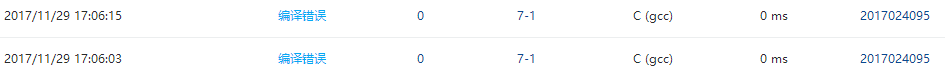要求三：
1.git地址：

2.git截图：（1）本周你学习了哪些内容？

（2）那些是难点？1.表格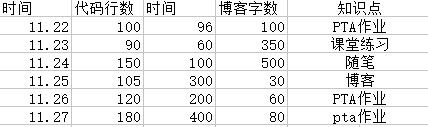2.折线图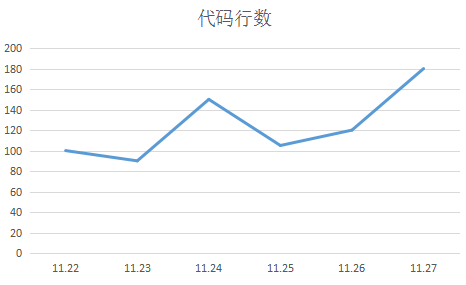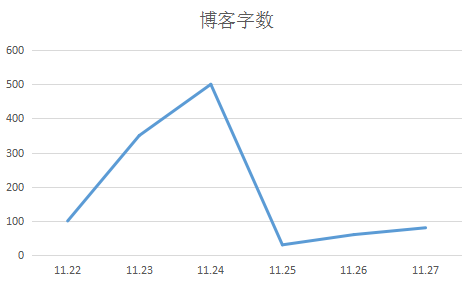posted @ 2017-12-03 17:42  张乐五班  阅读(488)  评论(10编辑  收藏  举报